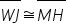Mathematics
Easy

Question

# What is the reason? Statement Reason WJ = MH Given_______

## ReflexiveSymmetricDefinition of congruenceDefinition of equalHint:

## The correct answer is: Definition of congruence

### Here , we have to find the reason of (WJ) ̅ ≅ (MH) ̅.Firstly, given is WJ = MH , Now, (WJ) ̅ ≅ (MH) ̅ , If lines are congruent then we use ≅ this symbol,Therefore, the reason of (WJ) ̅ ≅ (MH) ̅ is definition of congruence.The correct answer is definition of congruence.

In this question, we have to find the reason of (WJ) ̅ ≅ (MH) ̅. If the line are congruent then we also give then a sign of approximately equal ≅.

### Related Questions to study#### With Turito Foundation.#### Get an Expert Advice From Turito.